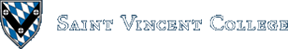Computing & Information Systems    DepartmentSearch CIS Site      Tutorials

## Software Design Using C++

### What is a Tree?

It is actually hard to define what we mean by a tree, but the idea can be seen in the following picture of an example tree showing course prerequisites. A course with a line to a second course below it is a prerequisite for the second course. The left to right ordering means nothing. We would still have the same tree if 330 were to the right of 310, for example.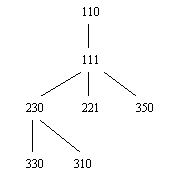At this point it is helpful to introduce some terminology. The root node (or simply root) is the node at the top of the tree diagram. In our case it is the one containing 110. Note that in computer science trees have their root at the top and branch out as you go down! This is simply the customary way of drawing trees.

The parent of a node is the one directly connected to it from above. In our example, 111 is the parent of 350, 230 is the parent of 310, 110 has no parent, etc. A child is a node connected directly below the starting node. Thus 350 is a child of 111, 310 is a child of 230, etc. It is simply the reverse of the parent relationship. Nodes with the same parent are called siblings. So, 221, 230, and 350 are siblings in our example.

An ancestor of a given node is either the parent, the parent of the parent, the parent of that, etc. In our example 110 is an ancestor of all of the other nodes in the tree. The counterpart of ancestor is descendant. For example, 310 is a descendant of 111, but 310 is not a descendant of 221.

The leaves of a tree (sometimes also called external nodes) are those nodes with no children. In our example, 221, 350, 330, and 310 are leaves. Note that leaves do not have to be at the lowest level in the tree. The other nodes of the tree are called non-leaves (or sometimes internal nodes).

Note that each node of a tree can be considered to be the root of a subtree consisting of that node and all its descendants. For example, the subtree rooted at 230 is as follows: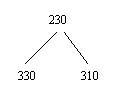A branch is a sequence of nodes such that the first is the parent of the second, the second is the parent of the third, etc. For example, in the above tree, the sequence 111, 230, 310 is a branch. The length of a branch is the number of line segments traversed (which is one less than the number of nodes). The above branch has length 2.

The height of a tree is the maximum length of a branch from the root to a leaf. The above tree has height 3, since the longest possible branch from root to leaf is either 110, 111, 230, 310 or 110, 111, 230, 330, both of which have length 3.

A more formal definition of a tree can be written as follows: A (general) tree consists of a set of nodes that is either empty or has a root node to which are attached zero or more subtrees. Of course, a subtree itself must be a tree. Thus this is a recursive definition. Note, too, that there is no left to right ordering of the subtrees.

### What is a Binary Tree?

A binary tree is similar to a tree, but not quite the same. For a binary tree, each node can have zero, one, or two children. In addition, each child node is clearly identified as either the left child or the right child. Thus there is a definite left-right ordering of the child nodes. Even when a node has only one child, that child must be identified as either the left child or the right child. As an example, here is the binary expression tree for the expression `4 * 5 - 3`. Note that a child drawn to the left of its parent is meant to be the left child and that a child drawn to the right of its parent is meant to be the right child.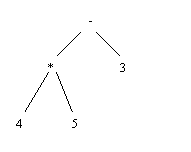Reversing the left to right order of any siblings gives a different binary tree. For example, reversing the subtrees rooted at `*` and at `3` gives the following different binary tree. It happens to be the binary expression tree for `3 - 4 * 5`.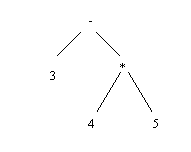### What is a Binary Search Tree?

A binary search tree is a binary tree in which the data in the nodes is ordered in a particular way. To be precise, starting at any given node, the data in any nodes of its left subtree must all be less than the item in the given node, and the data in any nodes of its right subtree must be greater than or equal to the data in the given node. Of course, all of this implies that the data items can be ordered by some sort of less than relationship. For numbers this can obviously be done. For strings, alphabetical ordering is often used. For records of data, a comparison based on a particular field (the key field) is often used.

The following is a binary search tree where each node contains a person's name. Only first names are used in order to keep the example simple. Note that the names are ordered alphabetically so that DAVE comes before DAWN, DAVID comes before DAWN but after DAVE, etc.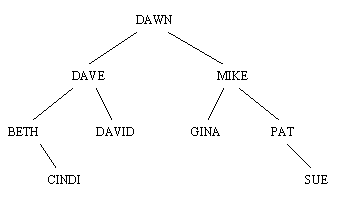How does one create a binary search tree in the first place? One way to do so is by starting with an empty binary search tree and adding the data items one by one. The first item becomes the root. The next item is placed in either a left child or right child node, depending on the ordering. The third item is compared to the root, we go left or right depending on the result of the comparison, etc. until we find the spot for it. In each case we follow a path from the root to the spot where we insert the new item, comparing the new item to each item in the nodes encountered along the way, going left or right at each node so as to maintain the ordering prescribed above.

### Traversals of Binary Trees

There are several well-known ways to traverse, to travel throughout, a binary tree. We will look at three of them. The first is an inorder traversal. This consists of three overall steps: traverse the left subtree (recursively), visit the root node, and traverse the right subtree (recursively). When we "visit" a node we typically do some processing on it, such as printing out the contents of the node. For example, an inorder traversal of the above binary search tree gives us the names in this order:

 `````` BETH CINDI DAVE DAVID DAWN GINA MIKE PAT SUE ``````

Note that we got the data back in ascending order. This will always happen when doing an inorder traversal of a binary search tree. In fact, a sort can be done this way. One first inserts the data into a binary search tree and then does an inorder traversal to obtain the data in ascending order. Some people call this a tree sort.

Now, how exactly did we get the above list of data? Essentially, we did so by following the recursive definition for an inorder traversal. First we traverse the left subtree of the root, DAWN. That left subtree is the one rooted at DAVE. How do we traverse it? By using the same three-step process. We first traverse its left subtree, the one rooted at BETH. Of course, we then have to go through the three steps on the subtree rooted at BETH. We begin by traversing its left subtree, but it is empty, so we visit the root, BETH. That is the first data item printed. Then we traverse the right subtree, the one rooted at CINDI. We use the three-step process on it, but since its subtrees are empty, we simply print the root, CINDI, which is the second item printed. We then back up to where we left off with the subtree rooted at DAVE. We have now traversed its left subtree, so we go on to print the root, DAVE, and then traverse the right subtree. Since the right subtree itself has empty subtrees, we end up just printing its root, DAVID. We continue in a similar fashion for the rest of this binary search tree.

The other two traversals that we will study are the preorder traversal and the postorder traversal. They are very similar to the inorder traversal in that they consist of the same three steps, but reordered slightly. The preorder traversal puts the step of visiting the root first. The postorder traversal puts the step of visiting the root last. Everything else stays the same. Here is an outline of the steps for all three of our traversals.
 Preorder traversal Visit the root Traverse the left subtree Traverse the right subtree Inorder traversal Traverse the left subtree Visit the root Traverse the right subtree Postorder traversal Traverse the left subtree Traverse the right subtree Visit the root
As an example, let's do a postorder traversal of the binary expression tree for `4 * 5 - 3` that we looked at earlier. The tree is shown again for convenience:First we traverse the left subtree of the whole binary tree. This is the subtree rooted at `*`. To do so, we apply our three steps. We traverse its left subtree, which results in printing `4`. Then we traverse the right subtree, which results in printing `5`. Then we visit the root, printing `*`. Next, we back up to where we left off with the whole binary tree. We have now traversed the left subtree, so we traverse the right subtree, printing `3`. Then we visit the root, printing `-`. Overall we end up printing `4 5 * 3 -`, the postfix form of the expression. A postfix expression is deciphered by looking at it left to right and using the fact that each operator (such as *) applies to the two previous values. Note that the traversal always works like this: a postorder traversal of a binary expression tree yields the postfix form of the expression. You may be familiar with postfix expressions in that some calculators use them. In ordinary mathematics we are used to using infix expressions, where operators such as + and * come between the two values to which they apply.

For practice try a preorder traversal of the same binary expression tree for `4 * 5 - 3`. The result should be `- * 4 5 3`. This is the prefix form of the expression, that is, the form in which each binary operator precedes the two quantities to which it applies. A preorder traversal of a binary expression tree always gives the prefix form of the expression.

The natural conjecture, then, would be that the inorder traversal of a binary expression tree would produce the infix form of the expression, but that is not quite true. With the above expression it is true. However, try the infix expression `(12 - 3) * 2`. Here parentheses are used to indicate that the subtraction should be done before the multiplication. The binary expression tree looks like this: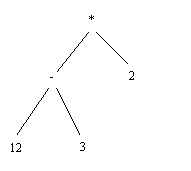As you can verify, an inorder traversal of this binary expression tree produces `12 - 3 * 2`, which is the infix form of a slightly different expression, one in which the multiplication is done before the subtraction. The problem is that we did not get the parentheses back. It is possible to modify the code for an inorder traversal so that it always parenthesizes things, but a plain inorder traversal does not give any parentheses.

### Uses of a Binary Search Tree

A binary search tree can be a very useful data structure. We have already seen that it can be used to create a sort routine. Such a sort routine is normally pretty fast. In fact, it is `Theta(n * lg(n))` on the average. However, it does have a bad worst case, namely when the data is already in ascending or descending order. (Try it. Start with a list of data items in order and insert them one by one into a binary search tree. What does this tree look like? Why would this make it slow to access the data later when we do the inorder traversal?)

Another use of a binary search tree is in storing data items for fast lookup later. In the average case it is pretty fast to insert a new item into a binary tree, because in the average case the data is fairly random and the binary tree is reasonably "bushy". (In such a tree it is known that the height of the binary tree is `Theta(lg(n))`, so that insertion is a `Theta(lg(n))` operation.) Similarly, doing a lookup of an item already in the binary tree follows the same pattern as used when it was inserted. Thus lookup is `Theta(lg(n))` on average.

For example, to look up GINA in the binary tree above, one compares GINA to DAWN, the root. Since GINA is larger, move to the right child, MIKE. Now compare GINA to MIKE. Since GINA is smaller, move to the left child GINA. Now compare GINA to the item in the node, also GINA, and we see that we have a match. All lookups are like this. One starts at the root and follows a path from the root to the matching item (or to a leaf if no match is ever found).

### A Pointer-Based Implementation of a Binary Search Tree

It is typical to create a binary search tree by using objects as the nodes and pointers to connect the nodes. This is the same approach that we used in implementing linked lists. The following files show the details: The `itemtype.h` file simply sets up `ItemType`, the type for the data that we want to store in our binary search tree. Then `bstnode.h` is used to set up the class for the node objects as shown below:

 `````` class BSTNodeClass { private: ItemType Info; BSTNodeClass * Left, * Right; public: BSTNodeClass(const ItemType & Item, BSTNodeClass * LeftPtr = NULL, BSTNodeClass * RightPtr = NULL): Info(Item), Left(LeftPtr), Right(RightPtr) { }; void GetInfo(ItemType & TheInfo) const; friend class BSTClass; }; typedef BSTNodeClass * BSTNodePtr; ``````

Each node has an `Info` field to hold a data item and two pointer fields, `Left` and `Right` for pointers to the left child and right child respectively. There is a constructor for the class with default values of `NULL` for the two pointer parameters. Note the use of the initialization list to copy `Item` into the `Info` field, `LeftPtr` into the `Left` field, etc. There is also a `GetInfo` function to extract the data from a node object. Finally, `BSTNodePTr` is set up as a type name for a pointer to one of these node objects.

The `bstnode.cpp` file is easy to follow, so let's move on to the `bstree.h` file. It sets up the class `BSTCLass` as shown here:

 `````` class BSTClass { private: BSTNodePtr GetNode(const ItemType & Item, BSTNodePtr LeftPtr = NULL, BSTNodePtr RightPtr = NULL); void FreeNode(BSTNodePtr NodePtr); void ClearTree(void); void ClearSubtree(BSTNodePtr Current); BSTNodePtr SubtreeFind(BSTNodePtr Current, const ItemType & Item) const; // The following are sometimes made protected instead of private: BSTNodePtr Root; int Count; public: // constructor: BSTClass(void); // destructor: ~BSTClass(void); int NumItems(void) const; bool Empty(void) const; void Insert(const ItemType & Item); // Some sort of Remove method could also be added, but // it would require effort to remake the binary search tree // after the deletion of a node. BSTNodePtr Find(const ItemType & Item) const; }; ``````

There are two data fields, `Count` and `Root`, both private. `Count` is used to keep track of how many items have been placed in the binary search tree. `Root` is the pointer to the root node of the binary search tree.

As for the class functions, there are private helping functions and the publicly available ones. The code for these is in the `bstree.cpp` file. The operations that are publicly available are: one to construct an empty binary search tree object, a destructor, a function to return the number of items in the binary search tree, a function to tell if the binary search tree is empty, a function to insert a new item so that we still have a binary search tree when finished, and a function to find an item in the binary search tree.

The private helping functions include one to manufacture a new node (containing a given data item and left and right pointers), a function to free up the space used by a node, a function to clear out the space used by the nodes of the binary search tree (leaving it empty), a function to free up the space used by a subtree, and a function to try to find a given item in a subtree. The latter function assists the public `Find` function in doing its job.

Now examine the code for the class functions. The constructor fills in a `NULL` as the `Root` and zero for the `Count`. This object represents an empty binary search tree. The destructor uses the `ClearTree` helping function to wipe out all of the nodes in the tree. As a destructor, the `BSTClass` object, with its `Root` and `Count` fields are automatically wiped out. Note the need to explicitly reclaim any space used outside of the `BSTClass` object as that is not handled automatically.

The `ClearTree` function works by calling the ```ClearSubtree ``` function on the subtree rooted at the overall root. This clears out all of the nodes in the binary search tree. Then the object is set to properly indicate an empty binary search tree.

The code for the `ClearSubtree` function is interesting and is therefore shown in detail below. Notice that it is handed a pointer to a node of the binary search tree and then follows a postorder traversal pattern to visit all of the nodes of the subtree rooted at the given node. Of course, we have two recursive calls to `ClearSubtree`. The stopping case for the recursion is whenever we have a `NULL` pointer for `Current`. In such a case there is nothing that has to be done to clear the subtree rooted there, as the subtree is empty!

 `````` void BSTClass::ClearSubtree(BSTNodePtr Current) { // Use a postorder traversal: if (Current != NULL) { ClearSubtree(Current->Left); ClearSubtree(Current->Right); FreeNode(Current); } } ``````

Why did we use a postorder traversal and not one of the other traversals? Postorder is used because we can only get rid of a root node after we have gotten rid of all of the nodes in its subtrees. If we got rid of a root node before we got rid of one of its descendants, we would be likely to lose any way to reach this descendant via our pointers.

The `GetNode` function uses the constructor for the `BSTNodeClass` to create a new node. Then it checks that the dynamic memory allocation for this node worked. This is exactly the procedure we followed when we implemented linked lists.

The next several class functions are very simple, so let's jump down to the `Insert` function. Its code is pasted in below:

 `````` void BSTClass::Insert(const ItemType & Item) { BSTNodePtr Current, Parent, NewNodePtr; Current = Root; Parent = NULL; while (Current != NULL) { Parent = Current; if (Item < Current->Info) Current = Current->Left; else Current = Current->Right; } NewNodePtr = GetNode(Item, NULL, NULL); if (Parent == NULL) Root = NewNodePtr; else if (Item < Parent->Info) Parent->Left = NewNodePtr; else Parent->Right = NewNodePtr; Count++; } ``````

This function uses a pair of pointers, `Current` which points at the node currently being examined, and `Parent` which points at its parent. These are advanced via a loop that does the appropriate comparison of the item to be inserted with the item at the current node, moving left or right as appropriate. The loop stops when `Current` is `NULL` and `Parent` points to the node under which to place the new one. A new node containing the item is then manufactured and linked in under this parent, either as the left child or the right child, as appropriate. Of course, there is a special case when `Parent` is also `NULL`. This means that you are inserting into an empty tree and just need to have the `Root` field of the binary search tree object point to the newly manufactured node.

The `Find` function just uses `SubtreeFind`, starting at the root of the overall binary search tree. `SubtreeFind` is written as a recursive function and is shown below for further examination:

 `````` BSTNodePtr BSTClass::SubtreeFind(BSTNodePtr Current, const ItemType & Item) const { if (Current == NULL) return NULL; else if (Item == Current->Info) return Current; else if (Item < Current->Info) return SubtreeFind(Current->Left, Item); else return SubtreeFind(Current->Right, Item); } ``````

The first two cases in this function are the stopping cases for the recursion. If the `Current` pointer is `NULL`, then we are searching an empty tree and the result is that the item cannot be found. This is shown by returning a value of `NULL`. If we have a match, we return a pointer to the current node. If the value that we are looking for is less than the one in the current node, we then search (recursively) this node's left subtree. Similarly, if the value that we are looking for is greater than the one in the current node, we then search this node's right subtree.

Finally, the `bsttest.cpp` file contains a test program for one of our binary search tree objects. It simply inserts a few items into the tree, tries to find a few values in the tree, etc.

### A Table Based on a Binary Search Tree

In much the same way that we created a list-based table, we can create a table that is based on a binary search tree. Both data structures allow us to insert data and retrieve it later. Once again, the new class (`BSTTableClass`) is derived by inheritance from the abstract base class `RamTableBaseClass`. The main change, of course, is that a `BSTTableClass` object contains a `BSTClass` object (a binary search tree), not a `ListClass` object (a linked list). Look through the following files to see the details. The following drawing shows the relationships between the classes used in the design of the above software. The drawing also includes those classes used in the list-based table that was designed earlier, as both the list-based table and the binary search tree type of table are derived from the same abstract base class.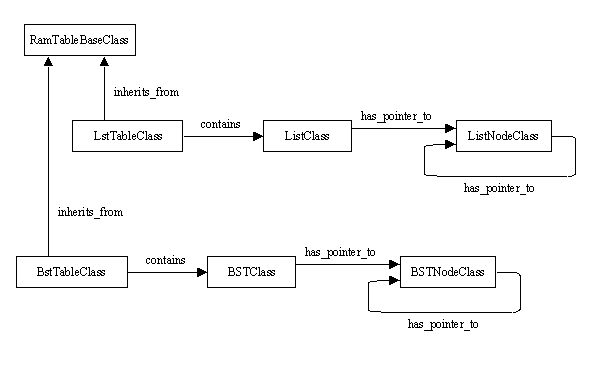### Related Items:

 PointersIntermediate Topic. A List-Based Table Heaps and Heapsort B-Trees AVL Trees Maps (in STL)
Back to the main page for Software Design Using C++

Author: Br. David Carlson with contributions by Br. Isidore Minerd
Last updated: July 30, 2015
Disclaimer